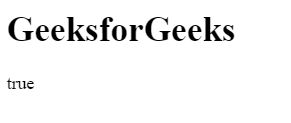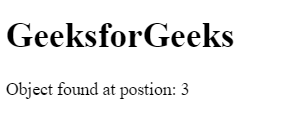Related Articles

# How to check if an array includes an object in JavaScript?

• Last Updated : 20 Jul, 2021

There are various methods to check an array includes an object or not.
Using includes() Method: If array contains an object/element can be determined by using includes() method. This method returns true if the array contains the object/element else return false.
Syntax:

`array.includes( element/object, startingPosition )`

Example:

Hey geek! The constant emerging technologies in the world of web development always keeps the excitement for this subject through the roof. But before you tackle the big projects, we suggest you start by learning the basics. Kickstart your web development journey by learning JS concepts with our JavaScript Course. Now at it's lowest price ever!

## html

 ```<``html``>`` ` `<``head``>``    ``<``title``>``        ``JavaScript includes() Method``    `````` ` `<``body``>`` ` `    ``<``h1``>GeeksforGeeks``     ` `    ``<``p` `id``=``"geeks"``>`` ` `     ` `    ````    ``<``script``>``        ``var obj = {"geeks1":10, "geeks2":12}``        ``var gfg = ["geeks1", "geeks2", obj];``         ` `        ``/* Use JavaScript includes() method */``        ``var num = gfg.includes(obj, 0);``         ` `        ``document.getElementById("geeks").innerHTML``                    ``= num;``    `````` ` `        `

Output:Using some() Method: The some() method uses the function for its evaluation and it executes the function once for each element present in the array. If it finds the object/element in the array then it returns true and stop the execution for remaining elements otherwise return false.
Syntax:

`array.some( function(currValue, arrIndex, arrObj), this )`

Example:

## html

 ```<``html``>`` ` `<``head``>``    ``<``title``>``        ``JavaScript includes() Method``    `````` ` `<``body``>`` ` `    ``<``h1``>GeeksforGeeks``     ` `    ````    ``<``script``>``     ` `        ``/* Declare an array */``        ``var arr = ["geeks1", "geeks2", "geeks3",``                    ``{1:"geeks4", 2:"geeks5"}];``                     ` `        ``var boolVar = arr.some( ``            ``value => { return typeof value == "object" } );``             ` `        ``document.write(boolVar);``    `````` ` `                    `

Output:Using filter() Method: The filter() method creates the array of all those elements/objects that passes the checking condition.
Syntax:

`array.filter( function(currValue, arrIndex, arrObj), this )`

Example:

## html

 ```<``html``>`` ` `<``head``>``    ``<``title``>``        ``JavaScript includes() Method``    `````` ` `<``body``>`` ` `    ``<``h1``>GeeksforGeeks``     ` `    ``<``p` `id``=``"geeks"``>`` ` `     ` `    ````    ``<``script``>``        ``var obj = {"geeks1":10, "geeks2":12}``        ``var arr = ["geeks1", "geeks2", "geeks3", obj];``         ` `        ``if(arr.filter(value=> value==obj).length > 0)``            ``document.write("true");``        ``else``            ``document.write("false");``    `````` ` `                    `

Output:Using findIndex() Method: The findIndex() method returns the position of searched object/element if it present in the array and stop the execution for rest elements. If the element/object not found then return -1.
Syntax:

`array.findIndex( function(currValue, arrIndex, arrObj), this )`

Example:

## html

 ```<``html``>`` ` `<``head``>``    ``<``title``>``        ``JavaScript includes() Method``    `````` ` `<``body``>`` ` `    ``<``h1``>GeeksforGeeks``     ` `    ````    ``<``script``>``     ` `        ``/* Declare an array */``        ``var arr = ["geeks1", "geeks2", "geeks3",``                ``{"geeks1":10, "geeks2":12}];``                 ` `        ``var num = arr.findIndex( ``            ``value => { return typeof value == "object" } );``             ` `        ``document.write("Object found at position: " + num);``    `````` ` `                    `

Output:JavaScript is best known for web page development but it is also used in a variety of non-browser environments. You can learn JavaScript from the ground up by following this JavaScript Tutorial and JavaScript Examples.

My Personal Notes arrow_drop_up